Equivalent Width Spectroscopy Lab
Updated automatically every 5 minutes

Equivalent Width Spectroscopy Lab

# Searching for Europium using Stellar Spectroscopy

This activity allows students to explore how to find the relative abundance of a given element using the spectral features in starlight. The spectra used here were collected by a team of teachers in the summer of 2019 at McDonald Observatory using the Sandiford Echelle Spectrograph attached to the 2.1 meter Otto Struve Telescope.

These spectra are part of a larger project to collect data on evolved sun-like stars with low abundances of the lanthanide elements. For more information on this class of stars, see the original paper on the PASTEL catalog doi: 10.1051/0004-6361/201014247. You can also search the PASTEL catalog.

## Who are you?

Put your name (s), the date, and course information.

# Spectroscopy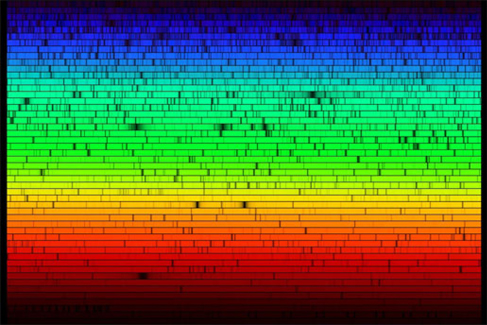Figure 1 - Visible light spectrum of the sun. (Fraknoi et al., 2017)

Light from objects in space can tell us a lot about the object. If we use that light to do spectroscopy, we can determine the temperature of the object, the nature, and speed of its motion, and we can find out what it's made of. We are exploring the chemical makeup of some stars in this project. If you need some background on what a spectrum is and what it can do for astronomers, check out this link.

## Question 1

How does a spectrometer or spectrograph turn starlight into colors? You can use the link above for a hint.

## The Sandiford Echelle Spectrograph

The spectra we are analyzing were collected using the historic Otto Struve Telescope at McDonald Observatory with the Sandiford Echelle Spectrometer.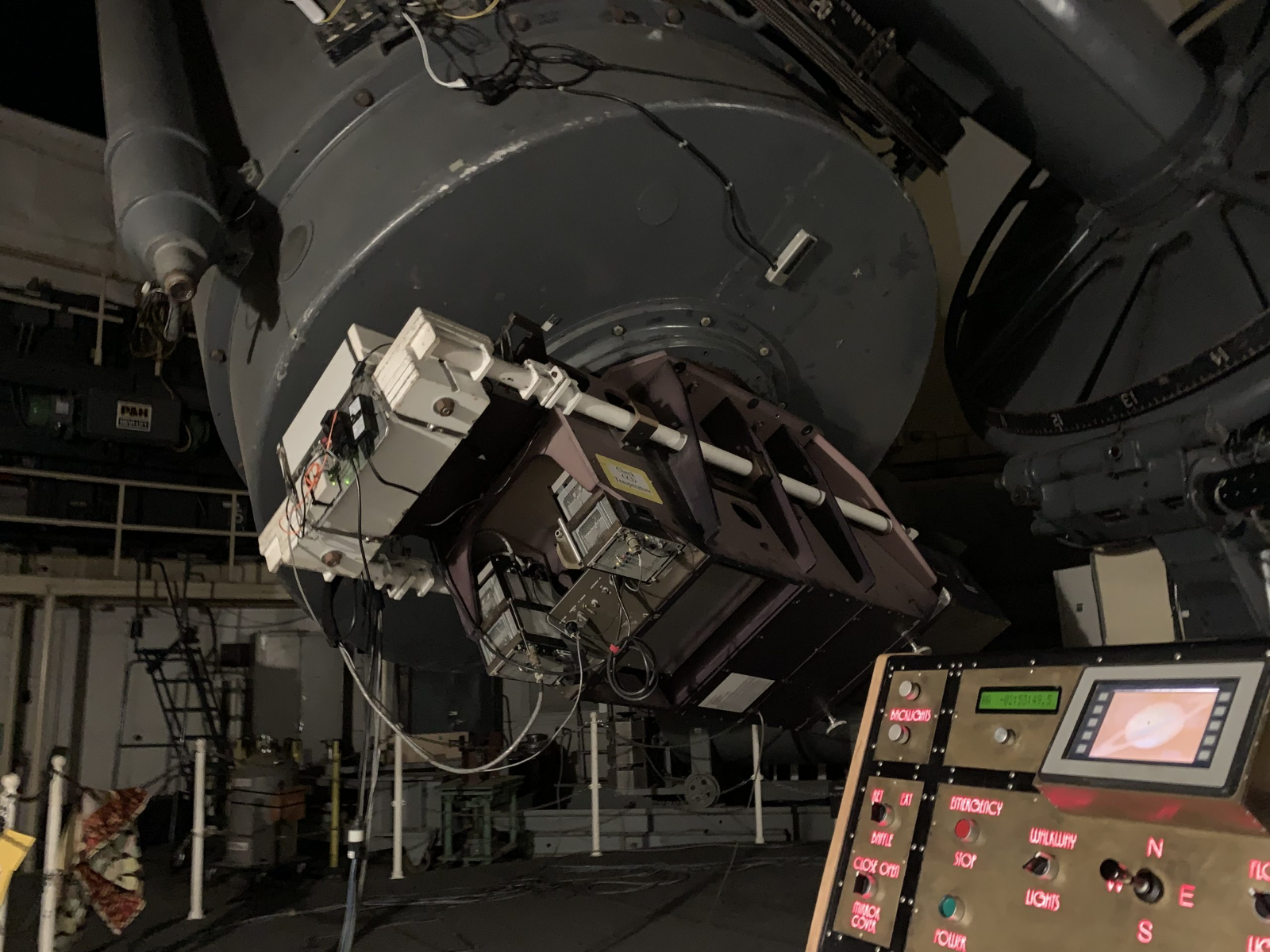Figure 2 - Sandiford Echelle Spectrograph at Cassegrain focus on Otto Struve Telescope, summer 2019.

## What is a spectrum?

This is the spectrum of a star. The y-axis represents the amount of light and the x-axis represents the wavelength of the particular feature. Spectroscopy can tell us about objects in space. For instance, using nothing more than the light from the star and some math, you can get a sense of the relative amount of a particular element in a star's atmosphere by looking at the absorption line. That is what we are doing today.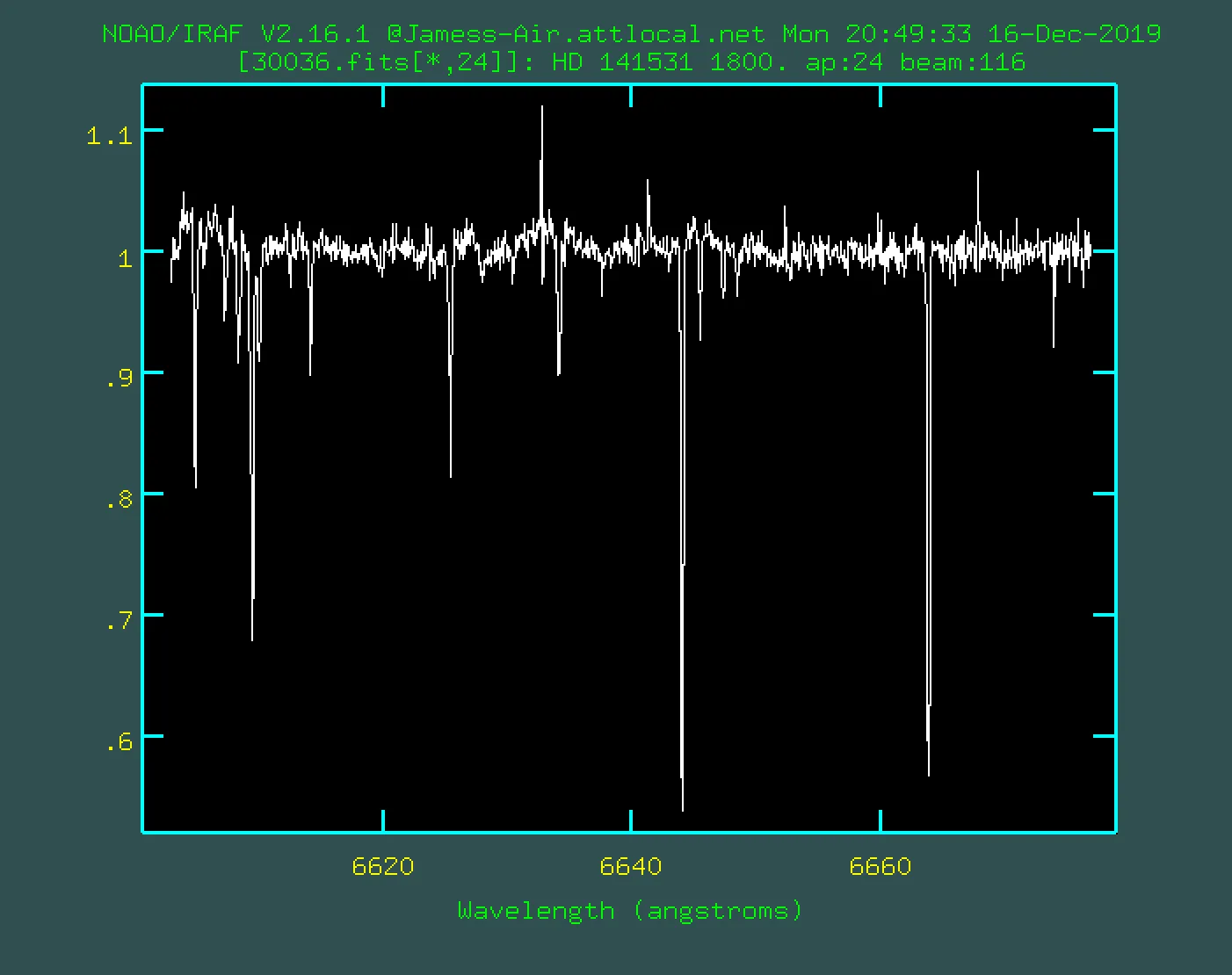Figure 3 - IRAF spectrum plot for star HD 141531 from summer 2019 observing run.

When one star has more of an element in its atmosphere than another, the absorption line or dips in the light will be deeper because those atoms took some of the light leaving the star and absorbed it so those photons won't make it to us.

For our data, the units are really strange. Flux is a unit of power which is energy per time unit. Here our flux is relative which means 1.0 would be the brightest part of the light from the star and the dips are where the light is less bright because it is being absorbed. Notice the brightness varies by wavelength. That is how we are able to look for the signature of particular elements. Specific elements absorb starlight at only specific wavelengths. The dips are the fingerprints of the element.

You will use data to analyze some absorption features for a few stars. We are looking for the atoms nickel and europium.

# Searching for Europium

Elements beyond lithium on the periodic table are produced by stars. Some of the heaviest elements come from the most awesome stellar explosions. Supernovae and kilonovae can make lots of heavy atoms all at once. After the debris from those explosions get swept up in new stars, these atoms can be found in the atmospheres of younger stars.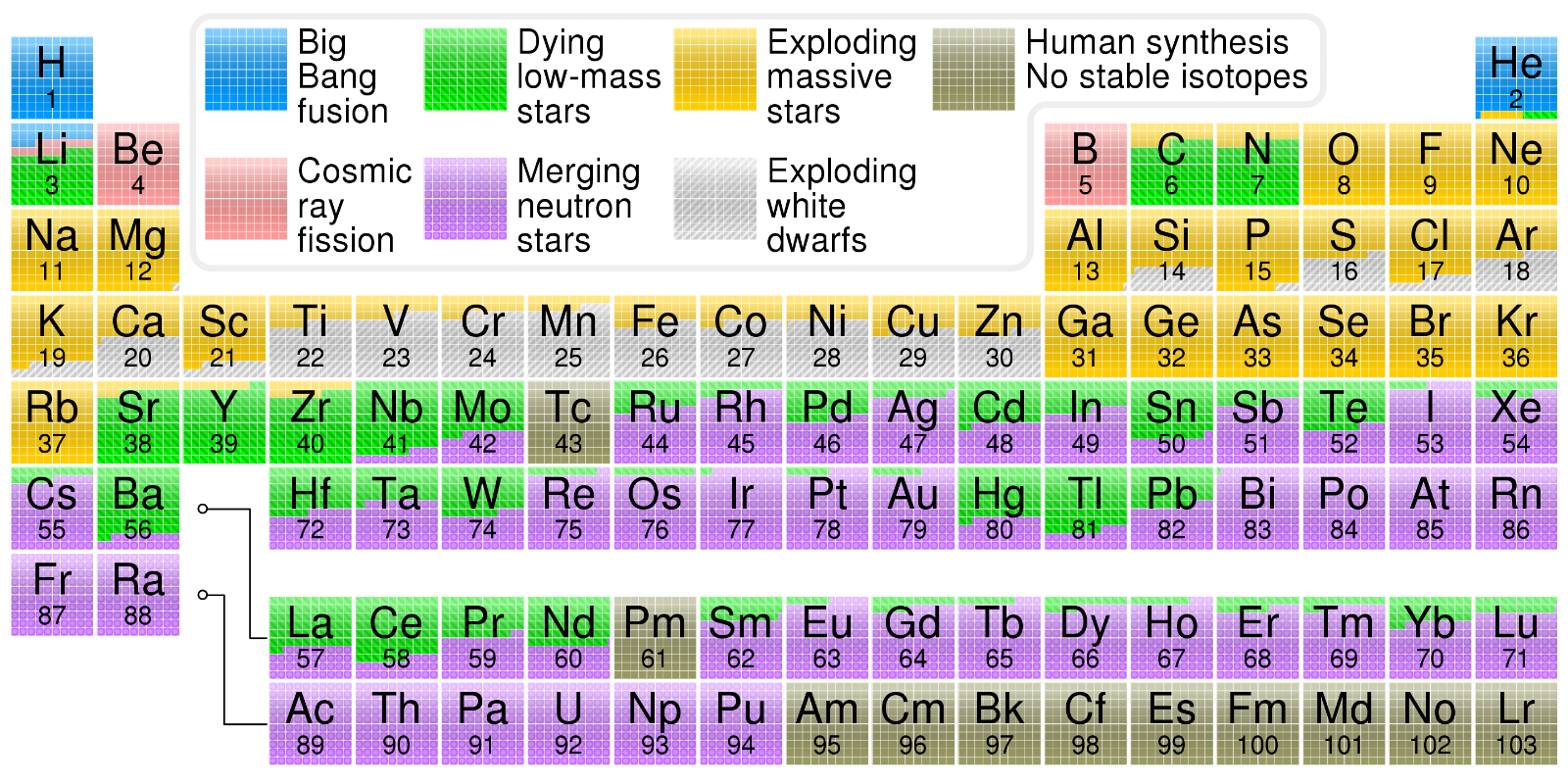Figure 4 - A stellar nucleosynthesis version of the periodic table of the elements. (Wikimedia, 2020)

These spectra we are analyzing here are part of a stellar survey looking for the presence of lanthanide elements. One lanthanide element, europium, has a signature that can be seen using the Sandiford-Echelle spectrograph.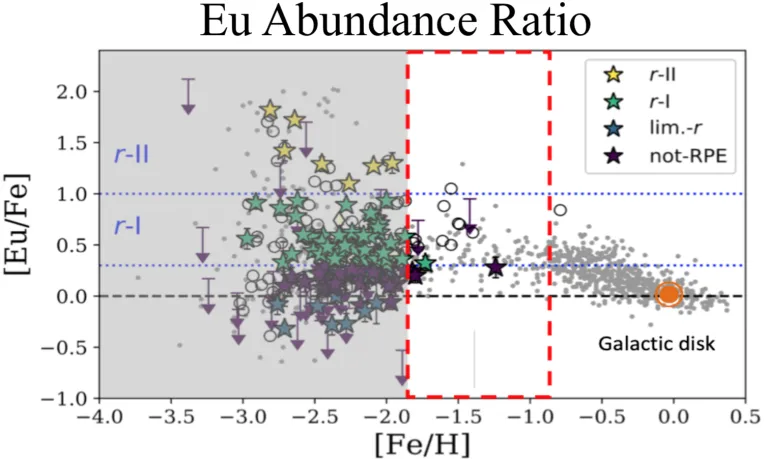Figure 5 - Metallicity constraints for stellar observational targets. (Sneden, 2019)

Five stellar spectra are stored in a Google spreadsheet and you are going to analyze and plot the data. You will compare how much europium these stars have by using the nickel absorption line as a measuring stick. Nickel is a common element among many stars and its signature is easily detectable.

Start by saving a copy of the data from the spreadsheet to your own Google Drive. If you are doing this as a group, make sure the person with the copy of the data shares access with everyone in the group so everyone can access the data.

Each sheet in the spreadsheet contains the data for one star.  Use the example for opening the first sheet to open all 5. When we plot a spectrum, the wavelength values (in angstroms - Å) are plotted along the x-axis and the flux or amount of light from the star is plotted along the y-axis.

## Question 2

If these spectra run from 6643 Å to 6646 Å, what color from the visible spectrum would the light appear? You can assume the visible spectrum runs from 300 nm to 700 nm. Note: 1 nm = 10 Å.

# Plotting A Stellar Spectrum

Watch the “HOW TO” video: https://www.youtube.com/watch?v=XkOUVOJrtrQ

2. Highlight the 2 columns of data. Most spreadsheets will assume the left column goes on the x-axis and the right column goes on the y-axis. This data is ready to go since column A is the wavelength in Angstroms and column B is the relative flux or amount of starlight. You can think of this as the brightness of a particular wavelength.
3. The easiest way to measure with the plot is if the chart type is set to ‘smooth line’.
4. Change the title of the graph to “Star name Spectrum” but use the real star name. :)
5. Change the y-axis title to Relative Flux or something similar.
6. Change the limits on the x-axis and y-axis so just the region with the absorption feature is visible.
7. Change the tick marks on the x-axis and y-axis so you can easily read off the plot. I used 0.05 on the y-axis with 4 minor marks and 0.1 on the x-axis with 4 minor marks. I also turned on all the various tick marks.
8. Do this process for each star.

## Question 3

What features do you see for the spectra? How many major features do you see? How are spectra similar and how are they different? Remember to describe how the plots are shaped relative to one another.

## Question 4

What do the dips represent? What does the wavelength of the lowest point in the dip represent? Hint: think how this is related to how a hydrogen atom can emit and absorb certain photons.

# Determining atomic abundance: Equivalent Width

We can measure how much of a particular element is in a star using spectroscopy. We plot the spectrum and find a particular absorption feature associated with a particular element. Then we can use a clever approximation to get a sense of the amount of the element present. The approximation is called the equivalent width. The equivalent width calculation is fairly simple geometry that comes from some very complicated stellar physics and chemistry.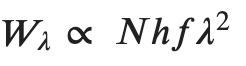Equation 1

The equivalent width Wλ varies as the number of atoms of that element, N. The product ℎ𝑓 is Planck's constant times the frequency of the absorption feature. And 𝜆 is the wavelength of the absorption feature. This strange notation is common for stellar spectroscopy.

## Making the EW rectangle: Full-Width at Half MaxFigure 6 - Example plot for determining the equivalent width for absorption feature.

In order to find the equivalent width, you'll need to find the full-width at half-max (FWHM). Notice the inverted bell-curve shape in the absorption line for Nickel. Since the curve is upside down for our absorption region, we need to find the minimum y-value. Our dip appears to go to around 0.45 below the top of the flux axis. If we divide by 2 we get the half-max of 0.225 below 1. You can see the blue flux curve must go through this y-value twice. Once on the left at around 6643.5 Å and again on the right at around 6643.75 Å. The EW is thenEquation 2

## How to measure equivalent width

1. First, count how far down from the y-axis the “bell curve” bit goes and write that in a cell labeled Max Abs or something similar. This is the maximum absorption for this feature.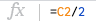2. Next, in an adjacent cell, hit = to enter a formula and click on the cell where you typed the value for the max absorption and divide that by 2. This is the half-max value.
3. We will use the half-max value to count down from 1 on the y-axis to where the curve meets that y-value on both the right and the left. Write down in an empty cell the left wavelength where the curve meets that half-max value and then write down in another empty cell the similar value on the right side of the curve.
4. Next, click an empty cell and click = to enter a formula. Be sure to put the difference between the right and left values in parentheses before you multiply by the max absorption.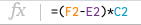5. This value represents the equivalent width for this spectral feature. This is a numerical way to describe the relative abundance of a particular atom for a star. (Note: this is not the actual number of a particular atom.)
6. Repeat for the other stars!

## Question 5

Choose a star. What is the equivalent width for the Ni I line near 6643 Å? You need to estimate the depth of the curve below 1. Then divide that by 2 and find the left and right wavelengths where the curve has that same flux below 1. Multiply these numbers. The units are Å since the flux here is relative and has no units.

• What star did you choose?
• What is the max absorption below 1?
• What is the half-max?
• At what 2 wavelengths does the flux curve pass through the half-max point?
• What is the equivalent width? (left wavelength - right wavelength)*(maximum absorption below 1)

# Stellar Nucleosynthesis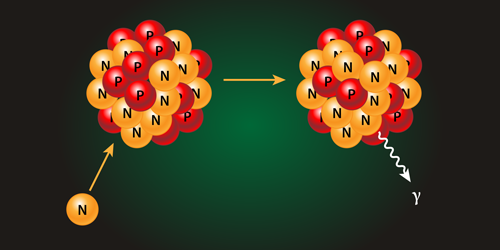Figure 7 - Artist conception of neutron capture event. (Stonebreaker, 2016)

Heavy elements (heavier than Fe) can be formed when neutrons smash into existing elements. The result is a leftover proton in the nucleus. Adding a proton to a nucleus means you changed from one atom to another. There are 2 types of stellar events that cause the rapid formation of elements: stellar explosions like a supernova and stellar mergers.

## Neutron Capture r-process & s-process

In 2017 astronomers observed a neutron star merger or kilonova. This event was so explosive and energetic that scientists observed the gravitational waves from the event. This type of merger is now thought to be the source of many heavy elements. Stellar explosions can cause rapid neutron capture or the r-process.

Sometimes a random neutron inside a star slams into a nucleus and makes a heavier element. This process is rare so it takes a long time to build up an element this way. That's why it's called the slow or s-process.

Europium is thought to mainly come from the r-process, although some europium comes from the s-process.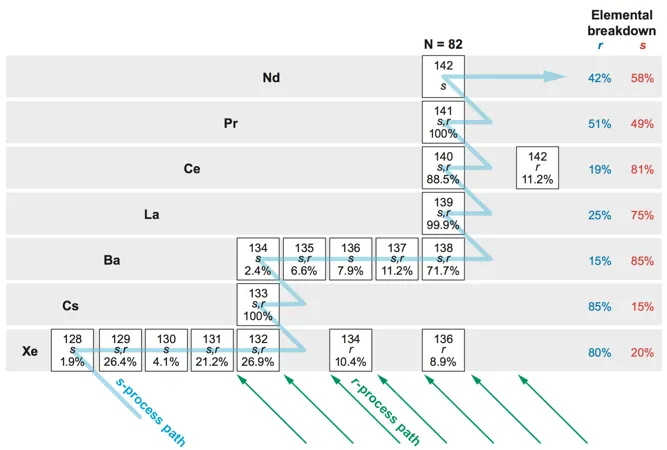Figure 8 - Neutron capture decay pathway through s-process for xenon. (Sneden et al., 2008)

## Question 6

If our target stars have comparable amounts of nickel, what measure should you use based on the results from the analysis? Rank the stars from most europium detected to least europium detected.

## Question 7

These stars all have very similar properties like mass and temperature. Why do they have varying amounts of europium? You should be able to list 3 physical processes by which europium atoms ended up in these stars.

# HR Diagram

Astronomers use the Hertzsprung-Russell Diagram to compare groups of stars and to look for patterns.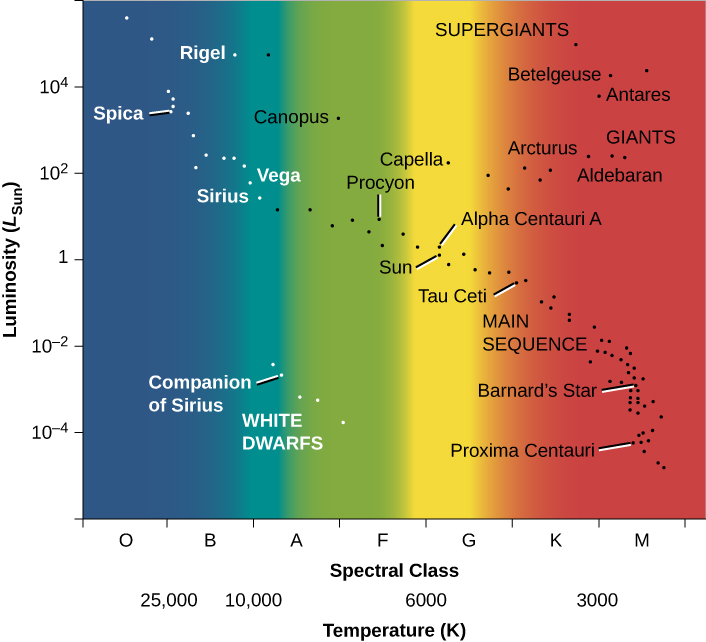Figure 9 - H-R diagram for a selected sample of stars. (Fraknoi et al., 2017)

Here is a classic example of an HR diagram with some of the known groupings labeled.

If you need a refresher on the HR diagram, take a look here.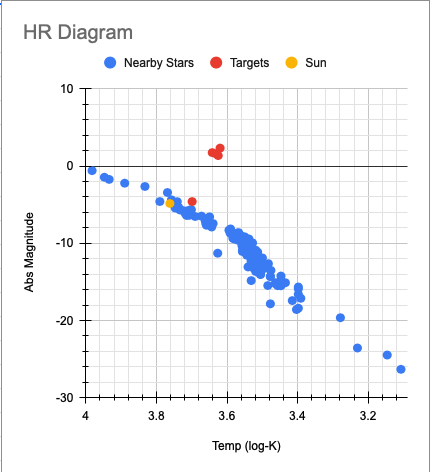Figure 10 - H-R diagram for nearby stars, the sun, and our stellar targets.

Here is an HR diagram of some of the nearest stars with our sun and our targets stars also plotted. The main sequence is the grouping of stars running from upper left to lower right and the red giant branch runs from the main sequence off to the upper right.

## Question 8

Using the HR diagram, can you put the results of the EW measurements in context? Are all the targets the same age? What role could the s-process have for these stars?

Remember that the log-log plot of a Hertzsprung-Russell diagram allows us to compare stars to known stars with known properties. Stars that are still powered mainly by hydrogen fusion are said to be on the main sequence. Stars not that different than the sun evolve off the main sequence into the red giant phase and eventually transition into white dwarf stars. The stars plotted on this HR diagram are nearby stars plus a few red giant stars which are farther away from us.

## Question 9 (optional)

Table 1 - Target Stars

 Targets logT (log K) logL (log W) HD 141531 3.63 30.1 HD 165195 3.64 30.3 TYC 5562-00446-1 3.63 29.9 TYC 5701-0019-1 3.70 23.9 V* SX Her 3.62 30.8

For this step, you are to plot the position on the given HR diagram for these 5 target stars. The logL is the y-axis and the logT is the x-axis. Like most HR diagrams, here the x-axis increases from right to left.

If you are plotting the targets using the spreadsheet: Open the HR Diagram spreadsheet and make a copy of it.  You should only put values in the color cells. Add a 3rd data series (logL) to the existing plot for your newly added target star data.

## Share and share alike!

This activity is meant to be shared. If you remix this work, let us know. Reach out for comments or questions. Written by Eileen Grzybowski, Justin Hickey, Olivia Kuper, and Jimmy Newland, Feb 2021.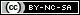References

Commons, W. (2020). File:Nucleosynthesis periodic table.svg --- Wikimedia Commons{,} the free media repository. https://commons.wikimedia.org/w/index.php?title=File:Nucleosynthesis_periodic_table.svg&oldid=483860915

Fraknoi, A., Morrison, D., Wolff, S. C., OpenStax College, & Rice University. (2017). Astronomy. OpenStax, Rice University. https://openstax.org/details/books/astronomy

Newland, J., Grzybowski, E., Hickey, J., Kuper, O., Sneden, C., & Finkelstein, K. (2020). Authentic Astronomical Research as Science Teacher Professional Development.